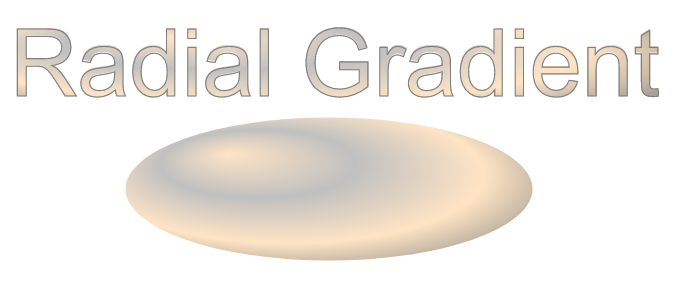# SVG Filters and Gradients – SVG code, Examples

Contents
[ Hide Show ]

## What is an SVG Filter?

SVG filters are widely used to add special effects to bitmaps or vector graphics. Filter effects are a way of a visual element’s treatment before displaying in the document. In other words, it is a set of operations to apply on an image can produce a wide variety of color and lighting effects, blurring, warping, noise and texture generation, etc. SVG filters are capable of creating complex effects in the browser and have the potential to be using in a variety of projects. They are supported by almost all modern browsers, including mobile ones.

Filter defines by `<filter>` element which sets within a `<defs>` element. It is never rendered itself and is conceptually described as the element that includes its children – filter primitives. The `<filter>` element has a set of attributes, here are some of them: x, y, width, height, filterUnits, primitiveUnits, core attributes, presentation attributes.

## SVG Filters

Each filter primitive makes a single graphical operation producing a visible result. The advantage of SVG filters is the ability to combine multiple types. The results obtained after applying one filter can be the source of the image for another filter.

Required attributes for filter primitive are x, y, width, and height. They set the area of the picture to which the filter will be applied. Each filter primitive can take one or two inputs and output only one result. The input data of the filter primitive is specified in the in attribute. The default is in=“SourceGraphic”. The output of the operation is defined in the result attribute.

Each filter requires a source image to processes. Otherwise, the filter will have nothing to render and so not work. All the filter primitives define in the SVG Filter specification.

## Gaussian Blur

The Gaussian blur function is obtained by blurring & smoothing an image using the Gaussian function. It can be considered as a filter that reduces image noise and minor details. Designers and photographers often apply Gaussian blur functions for different purposes: Gaussian blur can soften the image, it is useful for reducing chromatic aberration, it can hide a license plate or brand logo you don’t have permission to use, etc.

The `<feGaussianBlur>` filter creates a soft blur effect. It is a frequently used effect. The stdDeviation attribute specifies the number that characterizes the standard deviation for the blur operation. If two numbers are provided, the first number represents a standard deviation value along the x-axis of the coordinate system, the second one – on the y-axis.

Here is an example illustrates the various values of stdDeviation attribute ( gaussian-blur.svg):

`````` 1<svg height="400" width="600" xmlns="http://www.w3.org/2000/svg">
2    <defs>
3        <filter id="f1" x="-20" y="-20" height="100" width="100">
4            <feGaussianBlur in="SourceGraphic" stdDeviation="10" />
5        </filter>
6        <filter id="f2" x="-20" y="-20" height="100" width="100">
7            <feGaussianBlur in="SourceGraphic" stdDeviation="10, 0" />
8        </filter>
9        <filter id="f3" x="-20" y="-20" height="100" width="100">
10            <feGaussianBlur in="SourceGraphic" stdDeviation="0,10" />
11        </filter>
12    </defs>
13    <g stroke="none" fill="#20B2AA">
14        <ellipse cx="60" cy="80" rx="55" ry="35" />
15        <ellipse cx="200" cy="80" rx="55" ry="35" filter="url(#f1)" />
16        <ellipse cx="340" cy="80" rx="55" ry="35" filter="url(#f2)" />
17        <ellipse cx="500" cy="80" rx="55" ry="35" filter="url(#f3)" />
18    </g>
19</svg>``````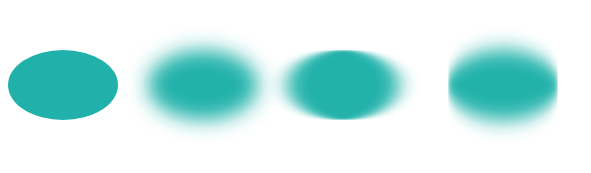The blur, added around a shape, often makes output picture larger than source one. Need to use negative numbers for x and y to avoid clipping the graphics added by the filter. In the example above, we made this in lines 3, 6 and 9.

You can create a Gaussian Blur effect in C# using Aspose.SVG for .NET API. To learn more about the Aspose.Svg.Filters namespace and consider detailed C# examples of using the `SVGFEGaussianBlurElement` class to apply the Gaussian Blur effect to SVG elements and bitmaps, please see the Gaussian Blur article.

A typical example of the drop shadow effect is to add a 3D look to a shape or text. Also, a drop shadow effect can be used to make an image stand out.

The `<feOffset>` filter primitive is used to offset a layer in SVG. In addition to the in and result attributes, this primitive accepts two main attributes – dx and dy, which define the distance the layer is offset along the x and y axes, respectively. The `<feBlend>` filter blends two objects; its mode attribute defines the blending mode.

`````` 1<svg height="200" width="200" xmlns="http://www.w3.org/2000/svg">
2    <defs>
3        <filter id="shadow" x="-20" y="-20" height="150" width="150">
4            <feOffset result="offset" in="SourceAlpha" dx="10" dy="10" />
5            <feGaussianBlur result="blur" in="offset" stdDeviation="10" />
6            <feBlend in="SourceGraphic" in2="blur" mode="normal" />
7        </filter>
8    </defs>
9    <ellipse cx="95" cy="90" rx="75" ry="55" fill="#20B2AA" filter="url(#shadow)" />
10</svg>``````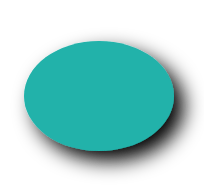Thee filters are used to create drop shadow effect:

1. `<feOffset>` takes `in="SourceAlpha"`, and shifts the result 10 px to the right and 10px to the bottom, and stores the result in the buffer as `"offset"`. Note, the alpha channel of the shape is used as input. The value `SourceAlpha` leads to a black-color result.

2. `<feGaussianBlur>` takes `in="offset"`, applies a blur of 10, and stores the result in a temporary buffer named `"blur"`.

3. `<feBlend>` takes two inputs `in="SourceGraphic"` and `in2="blur"` then blends the `SourceGraphic` on top of the offset black blurred image.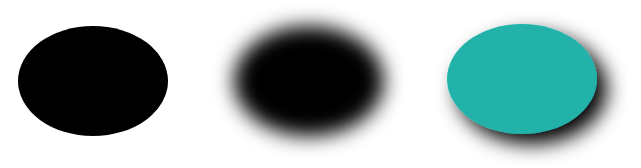You can create a drop shadow effect in C# using Aspose.SVG for .NET API. See the Drop Shadow Effect article to learn more about the Aspose.Svg.Filters namespace and consider detailed C# examples of applying the drop shadow effect to SVG shapes and text.

## SVG Light Effects

The lighting effect is making in SVG using a set of filters. Consider some of them: `<feDiffuseLighting>`, `<feSpecularLighting>`, and `<fePointLight>`. You can combine several filters, create and control the details for a lighting effect.

The `<fePointLight>` filter defines a light source which sets a point light effect. It can be used within the `<feDiffuseLighting>` or `<feSpecularLighting>` primitive as a child. Specific attributes x, y, and z indicate the position of the point light source. Both `<feDiffuseLighting>` and the `<feSpecularLighting>` filter light an image using its alpha channel as a bump map. The difference between them is the various calculations of the lighting model components.

Consider an example of a light effect ( light-effect.svg):

`````` 1<svg height="300" width="300" xmlns="http://www.w3.org/2000/svg">
2    <defs>
3        <filter id = "F1">
4            <feGaussianBlur in = "SourceAlpha" stdDeviation = "4" result = "blur" />
5            <feSpecularLighting result = "light" in = "blur" specularExponent = "25" lighting-color = "#bbbbbb">
6                <fePointLight x = "80" y = "60" z = "200" />
7            </feSpecularLighting>
8            <feComposite in = "SourceGraphic" in2 = "light" operator = "arithmetic" k1 ="0" k2 ="1" k3 ="1" k4 ="0" />
9        </filter>
10    </defs>
11    <g  fill = "INDIANRED" filter = "url(#F1)">
12        <circle cx="100" cy="100" r="60" />
13        <circle cx="100" cy="230" r="60" />
14        <circle cx="230" cy="100" r="60" />
15        <circle cx="230" cy="230" r="60" />
16    </g>
17</svg>``````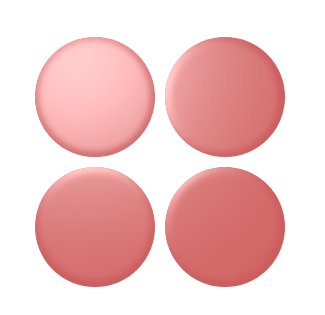In this example, four SVG filters are applied to create the light effect:

1. `<feGaussianBlur>` takes input `SourceAlpha`, which is the alpha channel of the source image. The result is stored in a temporary buffer named `"blur"`.

2. Lighting is done with the `<feSpecularLighting>` and `<fePointLighting>` filter effects. `<feSpecularLighting>` uses buffer `"blur"` as a model of a surface elevation and generates a lighting effect from a point source that sets in the `<fePointLighting>`filter. The result is stored in buffer `"light"`.

3. The `<feComposite>` filter takes two inputs `in = "SourceGraphic" in2 = "light"` and performs they combination using the arithmetic compositing operation. The output from the arithmetic operator for each result pixel is computed as: result = k1·in1·in2 + k2·in1 + k3·in2 + k4

The following is shown one more example of SVG filter effects combining ( button.svg):

`````` 1<svg height="200" width="200" xmlns="http://www.w3.org/2000/svg">
2    <defs>
3        <filter id="myF" x="-5" y="-5" height="100" width="150">
4            <feGaussianBlur in="SourceAlpha" stdDeviation="5" result="blur" />
5            <feOffset in="blur" dx="5" dy="5" result="offsetBlur" />
6            <feSpecularLighting in="offsetBlur" surfaceScale="8" specularConstant="0.7" specularExponent="2" lighting-color="#bbbbbb" result="specOut">
7                <fePointLight x="-100" y="-100" z="100" />
8            </feSpecularLighting>
9            <feComposite in="specOut" in2="SourceAlpha" operator="in" result="specOut" />
10            <feComposite in="SourceGraphic" in2="specOut" operator="arithmetic" k1="1.5" k2="0.5" k3="1" k4="0" result="litPaint" />
11            <feMerge>
12                <feMergeNode in="offsetBlur" />
13                <feMergeNode in="litPaint" />
14            </feMerge>
15        </filter>
16    </defs>
17    <ellipse cx="85" cy="70" rx="65" ry="45" fill="#20B2AA" filter="url(#myF)" />
18    <g fill="#696969" font-size="25" font-family="arial">
19        <text x="55" y="80">PUSH</text>
20    </g>
21</svg>``````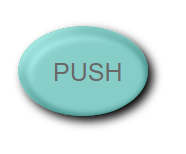In SVG Lighting Effects, you learn how to combine multiple filters to create and control SVG lighting effects in C# using the Aspose.SVG for .NET API.

## Color Filters

In SVG, you can create a wide variety of color effects that are supported in almost all modern browsers. When it comes to color handling, `<feColorMatrix>` is the best option. It is a type of color filter that uses a matrix to affect the color values for each RGBA channel. The `<feComponentTransfer>` is one of the most powerful SVG filter primitives. It gives control over the individual RGBA channels of the image, allowing you to create Photoshop-like SVG effects; for example, it can be used to posterize images.

Consider the main color filters – `<feColorMatrix>` and `<feComponentTransfer>`.

### `<feColorMatrix>` filter primitive

The `<feColorMatrix>` filter applies a matrix transformation to the RGBA channels of each pixel in the input image. As a result, a new set of color and alpha values is produced. In the common case, the matrix is written as an operation of the type attribute of the `<feColorMatrix>` filter. In the special cases of color matrices, the auxiliary operations of the type are used: saturate, hueRotate, luminanceToAlpha.

Let’s see examples of the saturate operation use ( saturation.svg):

``````1<svg width="640" height="480" viewbox="0 0 640 480" xmlns="http://www.w3.org/2000/svg">
2    <defs>
3        <filter id="myFilter">
4            <feColorMatrix in="SourceGraphic" type="saturate" values="0"></feColorMatrix>
5        </filter>
6    </defs>
7    <image filter="url(#myFilter)" href="https://docs.aspose.com/svg/images/drawing/park.jpg" width="100%" height="100%" />
8</svg>``````

The following figure is a series of images with the various saturate values: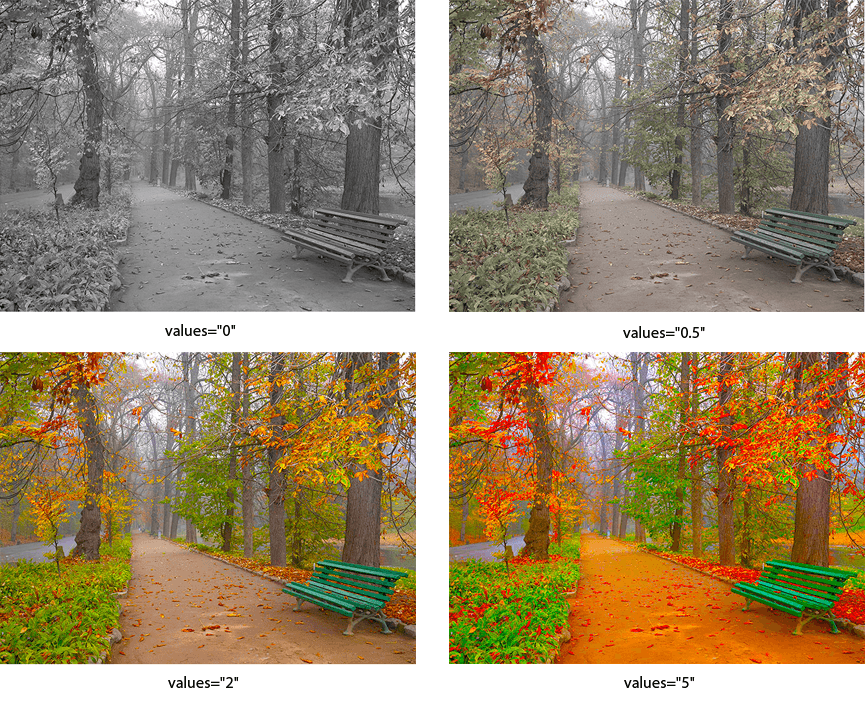The specific case of color matrices is image rotation along the color wheel. The following example illustrates using the hueRotate operation.

``````1<svg width="640" height="480" viewbox="0 0 640 480" xmlns="http://www.w3.org/2000/svg">
2    <defs>
3        <filter id="hueR">
4            <feColorMatrix in="SourceGraphic" type="hueRotate" values="40"></feColorMatrix>
5        </filter>
6    </defs>
7    <image filter="url(#hueR)" href="https://docs.aspose.com/svg/images/drawing/park.jpg" width="100%" height="100%" />
8</svg>``````

The following figure is a series of images with the various hueRotate values: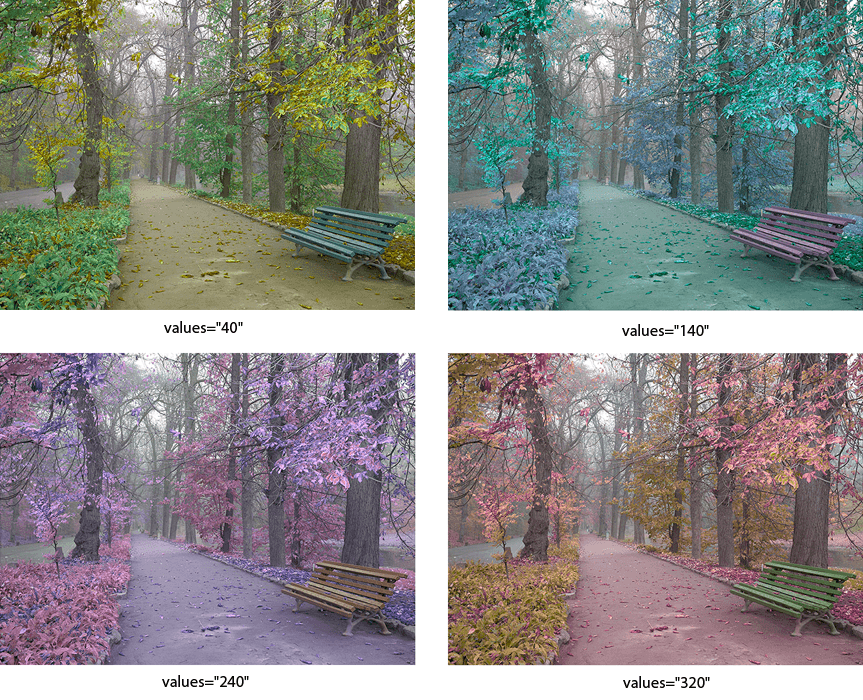### `<feComponentTransfer>` filter primitive

The `<feComponentTransfer>` filter allows you to perform linear, tabular, discrete operations with image channels, as well as change the gamma of each channel (RGBA). It lets operations like brightness adjustment, contrast adjustment, and color balance.

Let’s look at an example:

`````` 1<svg width="640" height="480" viewbox="0 0 640 480" xmlns="http://www.w3.org/2000/svg">
2    <defs>
3        <filter id="RGBA">
4            <fecomponenttransfer>
5                <feFuncR type="linear" slope="2.0" />
6                <feFuncG type="linear" slope="1.7" />
7                <feFuncB type="linear" slope="0.1" />
8                <feFuncA type="identity" />
9            </fecomponenttransfer>
10        </filter>
11    </defs>
12    <image filter="url(#RGBA)" href="https://docs.aspose.com/svg/images/drawing/park.jpg" width="100%" height="100%" />
13</svg>``````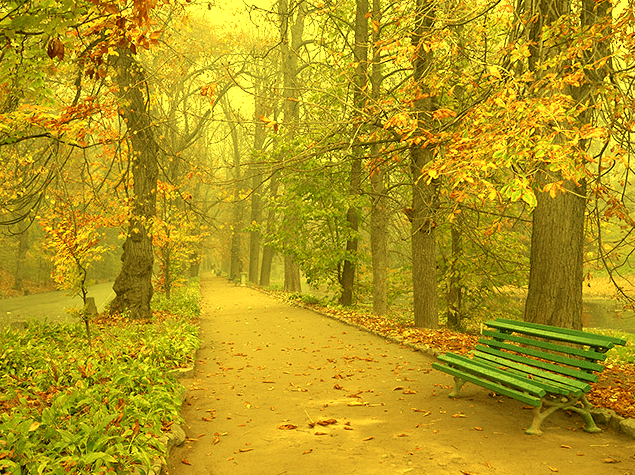The `<feComponentTransfer>` element allows modifying each of the R, G, B and A components present in a pixel. The RGBA components are also called transfer function elements: `<feFuncR>`, `<feFuncG>`, `<feFuncB>`, and `<feFuncA>`. In the type attribute, the type of function that allows modifying this component is determined. There are five function types: identity, table, discrete, linear, and gamma. More detailed information you find on the SVG Filter Effects page.

Read the Color Filters article if you want to create color filters in C#. You will learn about the `feColorMatrix` and `feComponentTransfer` filter primitives and look at C# code snippets that show how to use them.

A gradient is a smooth color transition between points on a drawing surface. There are two types of gradients defined by the following elements: `<linearGradient>` and `<radialGradient>`.

The `<linearGradient>` or `<radialGradient>` element must be embedded within a `<defs>` tag to promote reusability. The id attribute specifies a unique name for the SVG gradient. Other elements inside the file can reference it. The gradient can be applied for the fill or stroke properties for shapes, text, etc.

When talking about the SVG gradient, we have to define what a gradient vector is? The linear gradient vector connects starting and ending points onto which the gradient stops are mapped. The attributes x1, y1, x2 and y2 set the linear gradient vector. Their values can be either numbers or percentages.

The `<linearGradient>` has nested children `<stop>` elements that control the colors used in the gradient. Each color is specified with the `<stop>` tag. An offset attribute of the `<stop>` element indicates where the gradient stop is placed. For linear gradients, it represents a location along the gradient vector ( linear-gradient.svg).

`````` 1<svg height="250" width="700" xmlns="http://www.w3.org/2000/svg">
2	<defs>
4			<stop offset="10%" style="stop-color:LIGHTSALMON" />
5			<stop offset="50%" style="stop-color:TEAL" />
6			<stop offset="90%" style="stop-color:LIGHTPINK" />
8	</defs>
9    <ellipse cx="300" cy="170" rx="165" ry="55" fill="url(#grad1)" />
11</svg>``````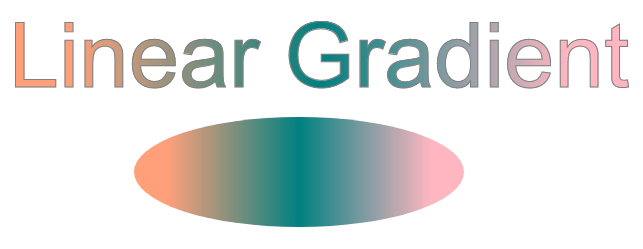In the example above, the linear gradient `id="grad1"` is referenced by the `<text>` and `<ellipse>` elements in the fill attribute. There are three `<stop>` nodes inside the linear gradient. In each of them, an offset attribute sets the position the SVG gradient gets a stop-color value.

A radial gradient is more difficult than a linear one. The colors change circularly rather than linearly in it. The attributes cx, cy, and r define the outermost circle for the radial gradient: cx and cy mark the center of this circle. Their values are specified as percentages of the width and height of a shape to fill. Both defaults to 50% if omitted. The fx and fy attributes define the innermost circle for the radial gradient. This is the point at which the SVG gradient «radiates».

In this example, the centers of the innermost and outermost border of the SVG gradient are the same ( radial-gradient.svg).

`````` 1<svg height="300" width="600" xmlns="http://www.w3.org/2000/svg">
2    <defs>
4            <stop offset="0%"   stop-color="BISQUE" />
7    </defs>
8    <ellipse cx="300" cy="170" rx="165" ry="55" fill="url(#myRG)" />
10</svg>``````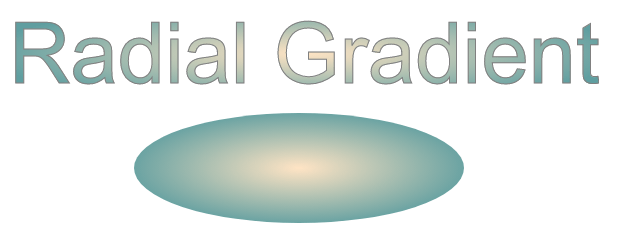Let’s see an example of the radial gradient, where the focal point (fx, fy) moved from the center of the object to position fx=25% and fy=25% ( radial-gradient1.svg):

`````` 1<svg height="300" width="600" xmlns="http://www.w3.org/2000/svg">
2    <defs>
4            <stop offset="0%" stop-color="BISQUE" />
5            <stop offset="30%" stop-color="SILVER" />
6            <stop offset="60%" stop-color="BISQUE" />
7            <stop offset="90%" stop-color="GREY" />
9    </defs>
10    <ellipse cx="300" cy="170" rx="185" ry="65" fill="url(#myRG)" fill-opacity="1" />